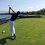# Division by infinityIt's a commonly known fact that one does not simply divide by zero. There are many mathematical proofs on the Internet and on Brilliant that are flawed due to dividing by zero, such as proving that 1+1=1. But if we aren't allowed to divide by 0, shouldn't we not be allowed to divide by infinity?

When you type in $0\times \infty$ on wolfram alpha, contrary to popular belief, the answer isn't 0, but rather it's indeterminate.image

let me show what I mean

$\dfrac{1}{\infty}=0$

$1=\infty \times 0$

Or

$\dfrac{2}{\infty}=0$

$2=\infty \times 0$

What... There are two results, but how can this be.

$1=\infty \times 0$

$\dfrac{1}{0}=\infty$

Or

$2=\infty \times 0$

$\dfrac{2}{0}=\infty$

So my question to you is "why is dividing by $\infty$ allowed but dividing by 0 is not)Note by Trevor Arashiro
5 years, 7 months ago

This discussion board is a place to discuss our Daily Challenges and the math and science related to those challenges. Explanations are more than just a solution — they should explain the steps and thinking strategies that you used to obtain the solution. Comments should further the discussion of math and science.

When posting on Brilliant:

• Use the emojis to react to an explanation, whether you're congratulating a job well done , or just really confused .
• Ask specific questions about the challenge or the steps in somebody's explanation. Well-posed questions can add a lot to the discussion, but posting "I don't understand!" doesn't help anyone.
• Try to contribute something new to the discussion, whether it is an extension, generalization or other idea related to the challenge.

MarkdownAppears as
*italics* or _italics_ italics
**bold** or __bold__ bold
- bulleted- list
• bulleted
• list
1. numbered2. list
1. numbered
2. list
Note: you must add a full line of space before and after lists for them to show up correctly
paragraph 1paragraph 2

paragraph 1

paragraph 2

[example link](https://brilliant.org)example link
> This is a quote
This is a quote
    # I indented these lines
# 4 spaces, and now they show
# up as a code block.

print "hello world"
# I indented these lines
# 4 spaces, and now they show
# up as a code block.

print "hello world"
MathAppears as
Remember to wrap math in $$ ... $$ or $ ... $ to ensure proper formatting.
2 \times 3 $2 \times 3$
2^{34} $2^{34}$
a_{i-1} $a_{i-1}$
\frac{2}{3} $\frac{2}{3}$
\sqrt{2} $\sqrt{2}$
\sum_{i=1}^3 $\sum_{i=1}^3$
\sin \theta $\sin \theta$
\boxed{123} $\boxed{123}$

Sort by:

There are 7 indeterminant forms namely,

$\frac{0}{0}, \frac{\infty}{\infty}, \infty - \infty, \infty \times 0, \infty^{0}, 0^{0}, 1^{\infty}$

here $0$, $1$ and $\infty$ are tending $0$,tending $\infty$ and tending 1

But

$\frac{exact 0}{exact 0}, \frac{tending0}{exact 0}, \infty + \infty, \infty \times \infty, \infty^{\infty}, \pm \infty$

Are undefined

Let me explain further

$exact 1^{\infty} = 1$

But

$tending 1 ^{\infty}$ is indeterminant

Another example

$\frac{exact 0}{tending 0} = 0$

But

$\frac{tending 0}{tending 0}$ is indeterminant

$\displaystyle exact0 \times \infty = 0$

But

$\displaystyle tending0 \times \infty$ is indeterminant

Ask anything if you don't understand because I copied the comment of mine when I explained same thing to other

- 5 years, 7 months ago

This is actually a great explaintion. If I understand, it's like using limits in a sense.

- 5 years, 7 months ago

One should understand the difference between these two situations:

$\Large a.$ When a number is $\textit {exactly}$ equal to some value &

$\Large b.$ When it $\textit {approaches from left or right}$ to some value.

In another way $0×\infty=0$ but $\textbf {(something infinitesimally small)×(something infinitely big)=undefined!! }$

- 5 years, 7 months ago

Exactly. Using limit sin x 0 value equals 0. Using limit e^x , value equals 1. Using limit e^1/x value equals infinite

- 4 years, 11 months ago